## Preliminaries

This vignette illustrates ‘metanetwork’ through a pyramid network example. The packages required to run the vignette are the following:

library(igraph)
library(metanetwork)

## Generating pyramid data set

### Generating the metaweb an representing it using ‘ggnet2’

We first generate a pyramid network using ‘igraph’ and represent it using ‘ggnet2’

library(igraph)
library(network)
library(intergraph)
library(GGally)

n = 5
#generate a lattice
g = igraph::make_lattice(dim = 2,length = n,directed = T)
#deleting nodes and edges
nodes_to_rm = c()
for (k in 1:(n-1)){
nodes_to_rm = c(nodes_to_rm,((k-1)*n+1):(k*n - k))
}
g = delete_vertices(g,nodes_to_rm)
g = delete_edges(g,c("7|12","8|13","9|14","2|5"))

## Building a metanetwork object

From the lattice metaweb and abundance table, build a S3 object of class ‘metanetwork’ using build_metanetwork

#building metanetwork object
meta0 = build_metanet(metaweb = g,abTable = presence)
class(g)
##  "igraph"
class(meta0)
##  "metanetwork"

method print prints a summary of the considered metanetwork.

print(meta0)
## metaweb has 15 nodes and 16 edges
## 3 local networks
## single resolution available

## Handling metanetworks

### the class ‘metanetwork’

A ‘metanetwork’ object consists in a list of ‘igraph’ objects:

• metaweb, the metaweb used to build the metanetwork, an ‘igraph’ object with node attribute $ab indicating the local relative abundance of each node and graph attribute $nameindicating "metaweb"
• local networks, a list of ‘igraph’ objects with node attribute $ab indicating the local relative abundance of each node in each network and graph attribute $name indicating local network names, that is rownames of the abundance table.
meta0$b$name
##  "b"
meta0$metaweb$name
##  "metaweb"
#abundances
table(V(meta0$b)$ab)
##
## 0.0833333333333333
##                 12
table(V(meta0$metaweb)$ab)
##
##  0.032258064516129 0.0645161290322581 0.0967741935483871
##                  3                  8                  4

Metaweb node relative abundances are the mean of the local relative abundances. Additional objects like abTable or trophicTable can be included in a ‘metanetwork’ object

### Computing trophic levels

‘metanetwork’ package enables 2D network representation with x-axis equals to trophic levels. To compute trophic levels, ‘metanetwork’ implements the method, based on Laplacian matrix, described in MacKay et al. 2020.
The metaweb needs to be connected for trophic levels to be unique. Local networks can however be disconnected (see Ref). A method compute_TL allows computing trophic levels and storing it as node attribute $TL of each network. #compute trophic levels for metaweb and local networks meta0 = compute_TL(meta0) V(meta0$metaweb)$name ##  "A" "B" "C" "D" "E" "F" "G" "H" "I" "J" "K" "L" "M" "N" "O" V(meta0$metaweb)$TL ##  0.000000e+00 0.000000e+00 1.000000e+00 4.440892e-16 1.000000e+00 2.000000e+00 4.440892e-16 1.000000e+00 2.000000e+00 3.000000e+00 ##  0.000000e+00 1.000000e+00 2.000000e+00 3.000000e+00 4.000000e+00 ## Representing metanetworks ‘metanetwork’ implements a layout algorithm, ‘TL-tsne’, specifically designed for trophic networks, based on trophic levels and on dimension reduction of graph diffusion kernel. ### Diffusion graph kernel Diffusion kernel is a similarity matrix between nodes according to a diffusion process. Let $$G$$ be a directed network, $$\mathbf{A}$$ its adjacency matrix and $$\mathbf{D}$$ its degree diagonal matrix. The laplacian matrix of $$G$$ is defined as: $\begin{equation} \mathbf{L} = \mathbf{D} - \mathbf{A} - \mathbf{A}^{T} \end{equation}$ The diffusion kernel is then defined as (Kondor & Lafferty, 2002): $\begin{equation} \textbf{K} = \exp(-\beta\mathbf{L}) = \sum_{k \geq 0} \frac{(- \beta\mathbf{L})^k}{k!} \end{equation}$ with $$\beta$$ a positive parameter. Diffusion kernel measures similarity between pairs of nodes by taking into account paths of arbitrary length. It does not restrict to direct neighbors. ### beta parameter $$\beta$$ is the single parameter of the diffusion kernel. It controls the weight given to the different paths in the diffusion kernel. It is also analogous to the diffusion constant in physics. We’ll see through examples its importance in squeezing networks. ### ggmetanet function The main metanetwork representation function is ggmetanet. It allows representing metaweb and local networks using ggnet and both layout algorithms. ggmetanet plots the metaweb of the current metanetwork by default. #ggmetanet# ggmetanet(metanetwork = meta0,beta = 0.1)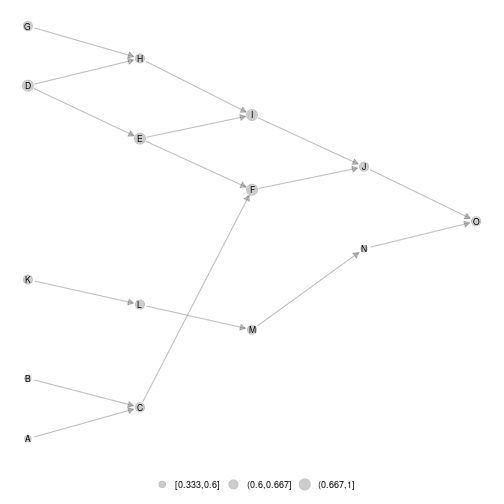plot of chunk unnamed-chunk-7 #ggmetanet# ggmetanet(metanetwork = meta0,beta = 0.45)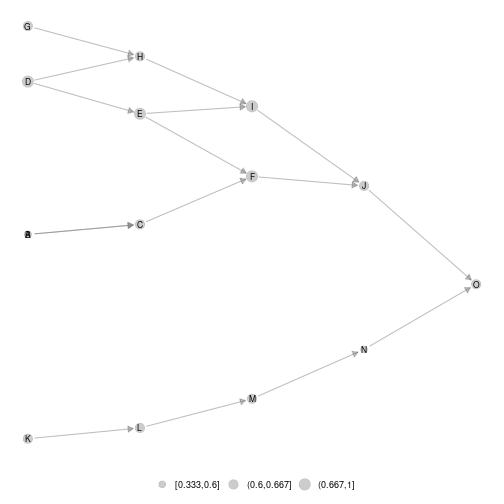plot of chunk unnamed-chunk-8 ggmetanet can also represent local networks (with specific layout) ggmetanet(g = meta0$b,beta = 0.1,metanetwork = meta0)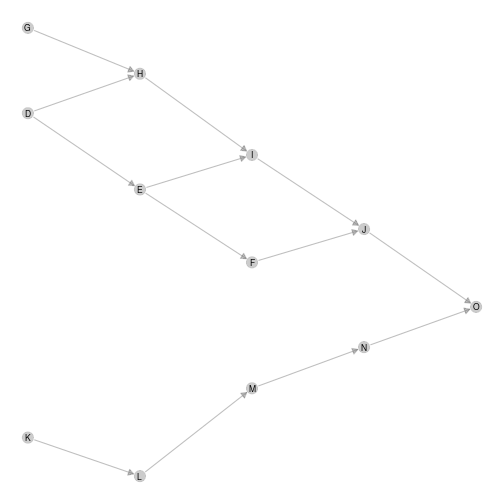plot of chunk unnamed-chunk-9

Increasing beta squeeze y-axis

ggmetanet(g = meta0$b,beta = 1,metanetwork = meta0)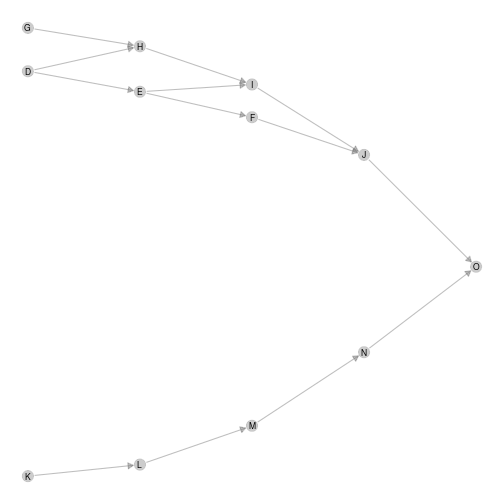plot of chunk unnamed-chunk-10 Moreover, it clusters nodes belonging to different ‘branches’. They become more and more similar when beta is increased. Representing disconnected networks If the metaweb needs to be connected, local networks can be disconnected due to sampling effects. In that case, trophic levels are computed using metaweb trophic levels. The basal species of each connected component has a trophic level equals to its value in the metaweb. ggmetanet(g = meta0$a,beta = 0.45,metanetwork = meta0)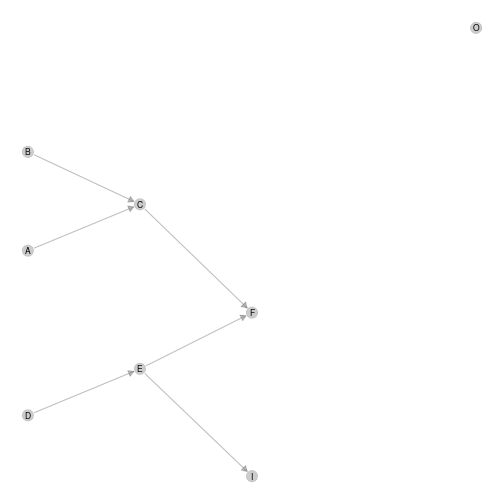plot of chunk unnamed-chunk-11

### diff_plot function

In order to compare local networks, a diff_plot function is implemented. It colors nodes according to their presence/absence or variation in abundance in both networks.

diff_plot(g1 = meta0$a,g2 = meta0$b,beta = 0.1,mode = 'TL-tsne',metanetwork = meta0)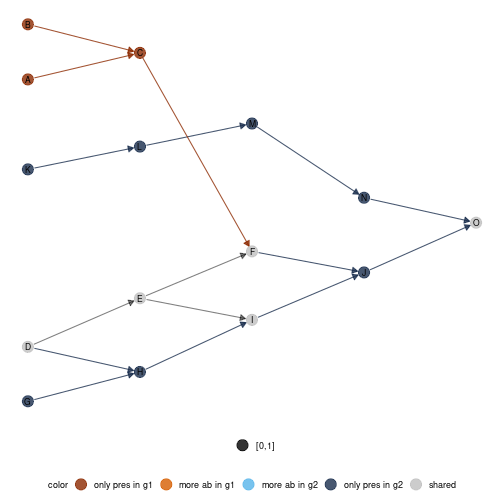plot of chunk unnamed-chunk-12

### Changing ggnet configuration parameters

In order to fine tune network plots, it is possible to modify ggnet parameters in metanetwork. An object ggnet.default is stored and wraps the different visualisation parameters. Change it to modify the plot.

ggnet.custom = ggnet.default
ggnet.custom$edge.size = 3*ggnet.default$edge.size
ggnet.custom$label.size = 7 ggmetanet(beta = 0.1,metanetwork = meta0, ggnet.config = ggnet.custom)plot of chunk unnamed-chunk-13 ### Attaching layout For network representation reproducibility and to gain computation time, we recommend to store ‘TL-tsne’ layout after computation. To do so, use the method attach_layout. Once the layout computed, it is attached to the network as node attribute. #for the metaweb meta0 = attach_layout(meta0,beta = 0.1) V(meta0$metaweb)$layout_beta0.1 ##  -10.5245709 0.5715783 -4.6297208 30.2718230 18.7743114 7.3023306 43.1058371 36.1900901 23.8856698 12.6402267 -44.7744167 ##  -38.1999701 -31.4062122 -24.7935220 -18.4134542 #for a local network meta0 = attach_layout(metanetwork = meta0,g = meta0$a,beta = 0.1)
V(meta0$metaweb)$layout_beta0.1
##   -10.5245709   0.5715783  -4.6297208  30.2718230  18.7743114   7.3023306  43.1058371  36.1900901  23.8856698  12.6402267 -44.7744167
##  -38.1999701 -31.4062122 -24.7935220 -18.4134542

Then, any call of ggmetanet or vismetaNetwork will use the computed layout for the desired $$\beta$$ value.

ggmetanet(meta0,beta = 0.1)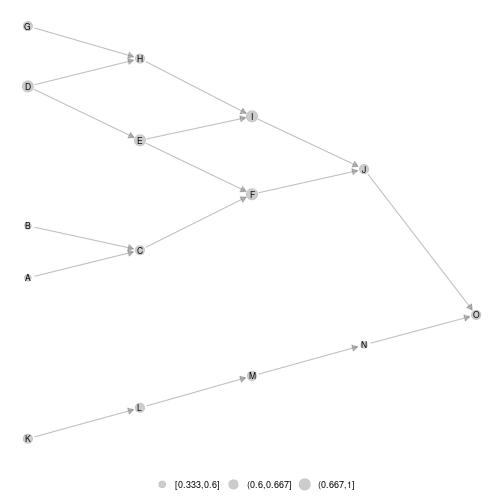plot of chunk unnamed-chunk-15

Using that way, network representation is reproducible.

#calling again ggmetanet
ggmetanet(meta0,beta = 0.1)Once the layout computed for the metaweb, it can be used to represent local network or difference network using layout_metaweb = T

#ggmetanet
ggmetanet(meta0,beta = 0.1,layout_metaweb = T)plot of chunk unnamed-chunk-17

ggmetanet(g = meta0$a,metanetwork = meta0,beta = 0.1,layout_metaweb = T)plot of chunk unnamed-chunk-17 ggmetanet(g = meta0$b,metanetwork = meta0,beta = 0.1,layout_metaweb = T)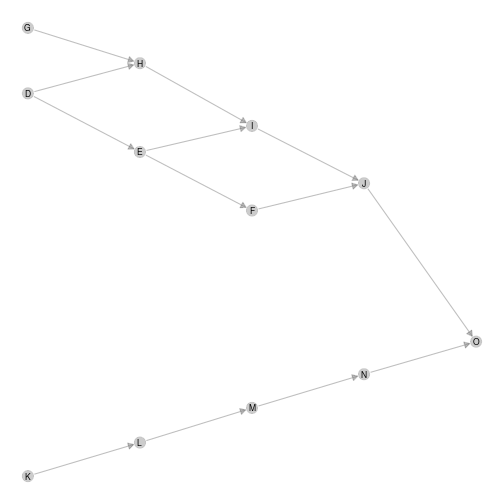plot of chunk unnamed-chunk-17

ggmetanet(g = meta0$c,metanetwork = meta0,beta = 0.1,layout_metaweb = T)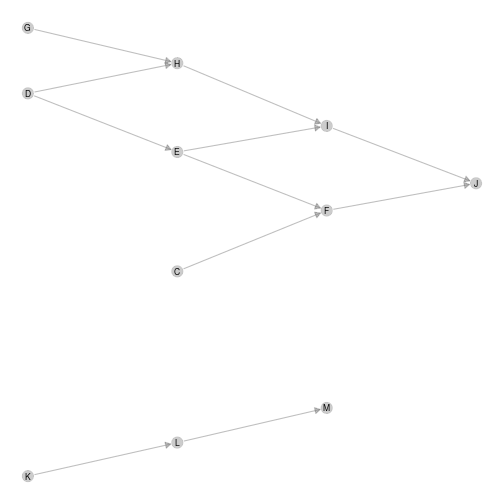plot of chunk unnamed-chunk-17 #diffplot diff_plot(meta0,meta0$a,meta0$b,beta = 0.1,layout_metaweb = T)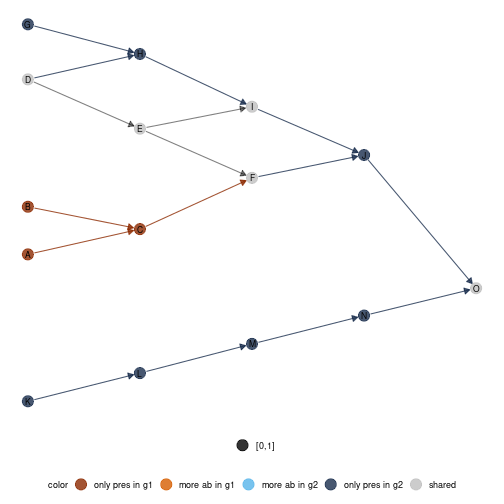plot of chunk unnamed-chunk-17 ## Computing network indices and metrics Besides network representation, ‘metanetwork’ package can compute usual network metrics (weighted connectance, mean and max trophic level, mean shortest path length). Network diveristy and dissimilarity indices based on Hill numbers are also implemented in order to quantitatively compare local networks.  #computing network metrics compute_metrics(meta0) ## connectance mean_TL max_TL mean_shortest_path_length ## metaweb 0.08428720 1.333333 4 2.069767 ## a 0.09375000 1.250000 4 1.400000 ## b 0.09027778 1.583333 4 1.968750 ## c 0.09090909 1.181818 3 1.550000 The metaweb is less connected than local networks but have the highest mean shortest path length. We now compute network diversity indices based on Hill numbers (cf. Ohlmann et al. 2019). The indices are based on node and link abundances are can be partitionned in $$\alpha$$-diversity, $$\beta$$-diversity and $$\gamma$$-diversity. A viewpoint parameter $$q$$ allows giving more weight (see compute_div documentation) #computing diversities compute_div(meta0) ##$nodes
## $nodes$Gamma
##  14.1607
##
## $nodes$mean_Alpha
##  10.18329
##
## $nodes$Beta
##  1.390582
##
## $nodes$Alphas
##  a  b  c
##  8 12 11
##
##
## $links ##$links$Gamma ##  14.53731 ## ##$links$mean_Alpha ##  9.502308 ## ##$links$Beta ##  1.529872 ## ##$links$Alphas ## a b c ## 6 13 11 Moreover, pairwise dissimilarity indices (both on nodes and links) are also implemented in metanetwork (see compute_dis documentation). #computing pairwise dissimilarities compute_dis(meta0) ##$nodes
##           a         b         c
## a 0.0000000 0.4942966 0.4699751
## b 0.4942966 0.0000000 0.1299762
## c 0.4699751 0.1299762 0.0000000
##
## c 0.5174744 0.1650477 0.0000000